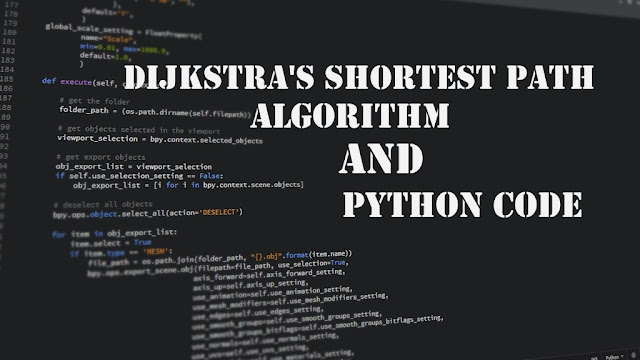## Posts

Showing posts from July, 2017

### Result TU : BE II/I All except BCE of 2073 Chaitra### Dijkstra's Shortest Path Algorithm and Its Python CodeThis algorithm finds the length of the shortest path from vertex a to vertex z in a connected, weighted graph. The weight of edge (i , j) is w(i , j) > 0 and the label of vertex x is L(x). At termination, L(z) is the length of the shortest path from a to z. Input: A connected, weighted graph in which all weights are positive; vertices a and z Output: L(z), the length of the shortest path from a to z Dijkstra(w,a,z,l)        L(a)=0         for all vertices x ≠ a             L(x)  =  ∞            T = set of all vertices        // T is the set of vertices whose shortest distance from a has      // not been found       while(z  ∈ T ) {            choose v ∈ T with minimum L(v)            T = T -{v}            for each x ∈ T adjacent to v                 L(x) = min{ L(x) , L(v) + w(v,x)}           } } NOTE: Algorithm From Discrete Mathematics By Richard Johnsonbaugh PYTHON CODE: 1 2 3 4 5 6 7 8 9 10 11 12 13 14 15 16 17 18 19 20 21 22 23 2